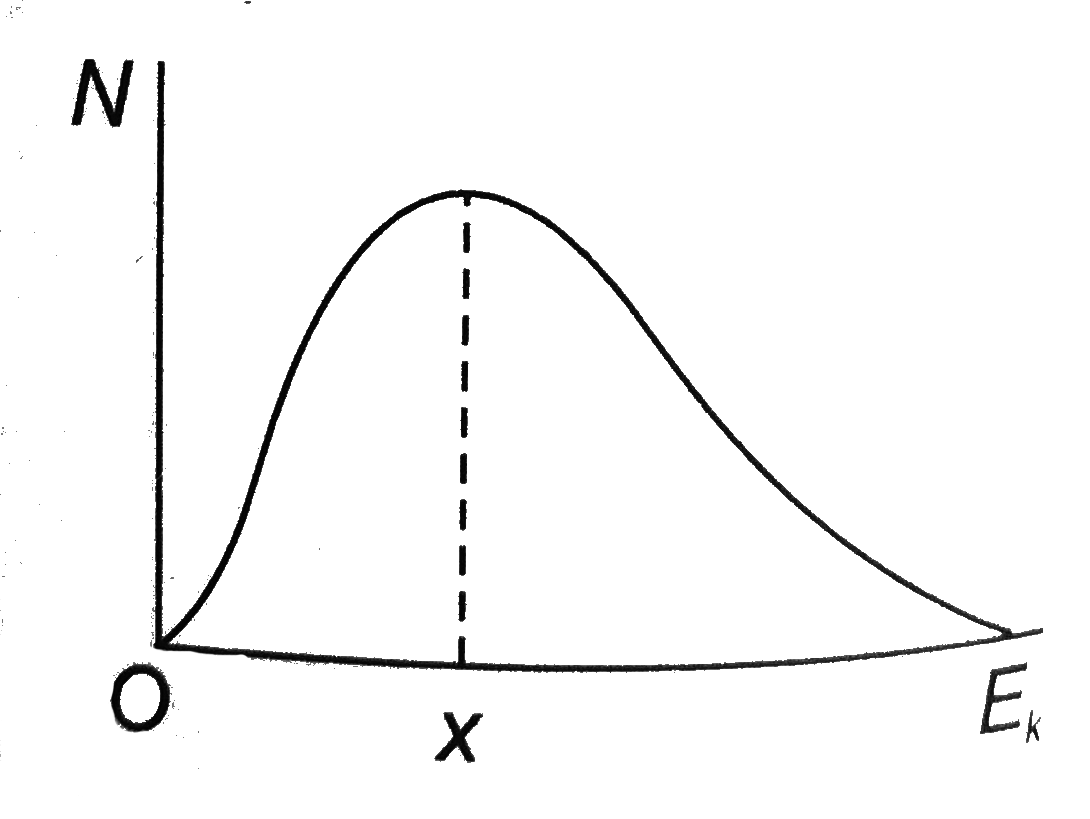# The adjoining graph shows the distribution of kinetic energies E_(k) among the consultant molecules of a gas at a uniform temperature. N is the nu

73 views
in Physics
closed
The adjoining graph shows the distribution of kinetic energies E_(k) among the consultant molecules of a gas at a uniform temperature. N is the number of molecules each having energy in a small energy band around E-(k). Which of the following statements is true?A. Provided that the temperature does not change, the kinetic energy of each molecules is fixed.
B. The commonest value of kinetic energy is also the greatest kinetic energy of any of the molecules.
C. The total kinetic of the molecules is independent of the temperature of the gas.
D. The value x of E_(k) at which the peak of the curve occurs increase when the temperature rises.

by (90.5k points)
selected

For (a), remeber that molecules are continually in collision with one another. For (b) look at the given graph carefully. For (d), remember that the root mean square speed increases with temperature increase.